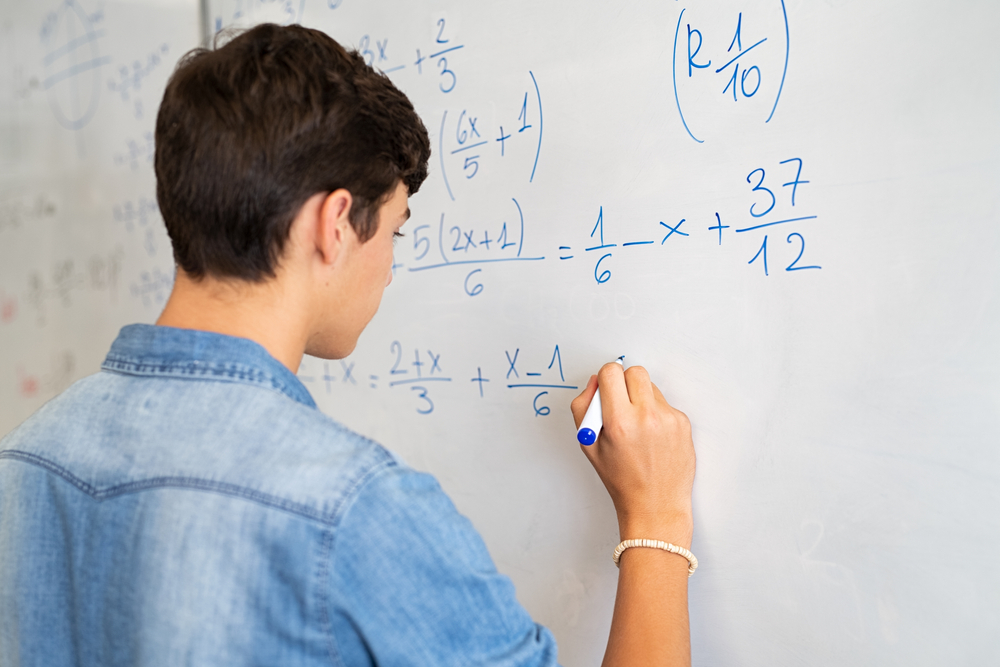# GCSE Edexcel Mathematics

Current Status
Not Enrolled
Price
Closed
Get Started
This course is currently closed

The GCSE Mathematics course consist of nineteen units. These are assessed in three examination sessions. The first examination sessions is a non calculator and the other two sessions at calculator papers. The main nineteen sections are :

Unit 1 Number
Unit 2 Algebra
Unit 3 Interpreting and representing data
Unit 4 Fractions, ratio and proportion
Unit 5 Angles and trigonometry
Unit 6 Graphs
Unit 7 Area and volume
Unit 8 Transformation and constructions
Unit 9 Equations and inequalities
Unit 10 Probability
Unit 11 Multiplicative reasoning
Unit 12 Similarly and congruence
Unit 13 More trigonometry
Unit 14 Further statistics
Unit 15 Equations and graphs
Unit 16 Circle theorems
Unit 17 More algebra
Unit 18 Vectors and geometric proof
Unit 19 Proportion and graphs

At The Arc Education, we assess each unit, identify gaps and bridge the gaps before certifying the completion of the course.

Hello,
How will you like to start your journey with Myrtle?